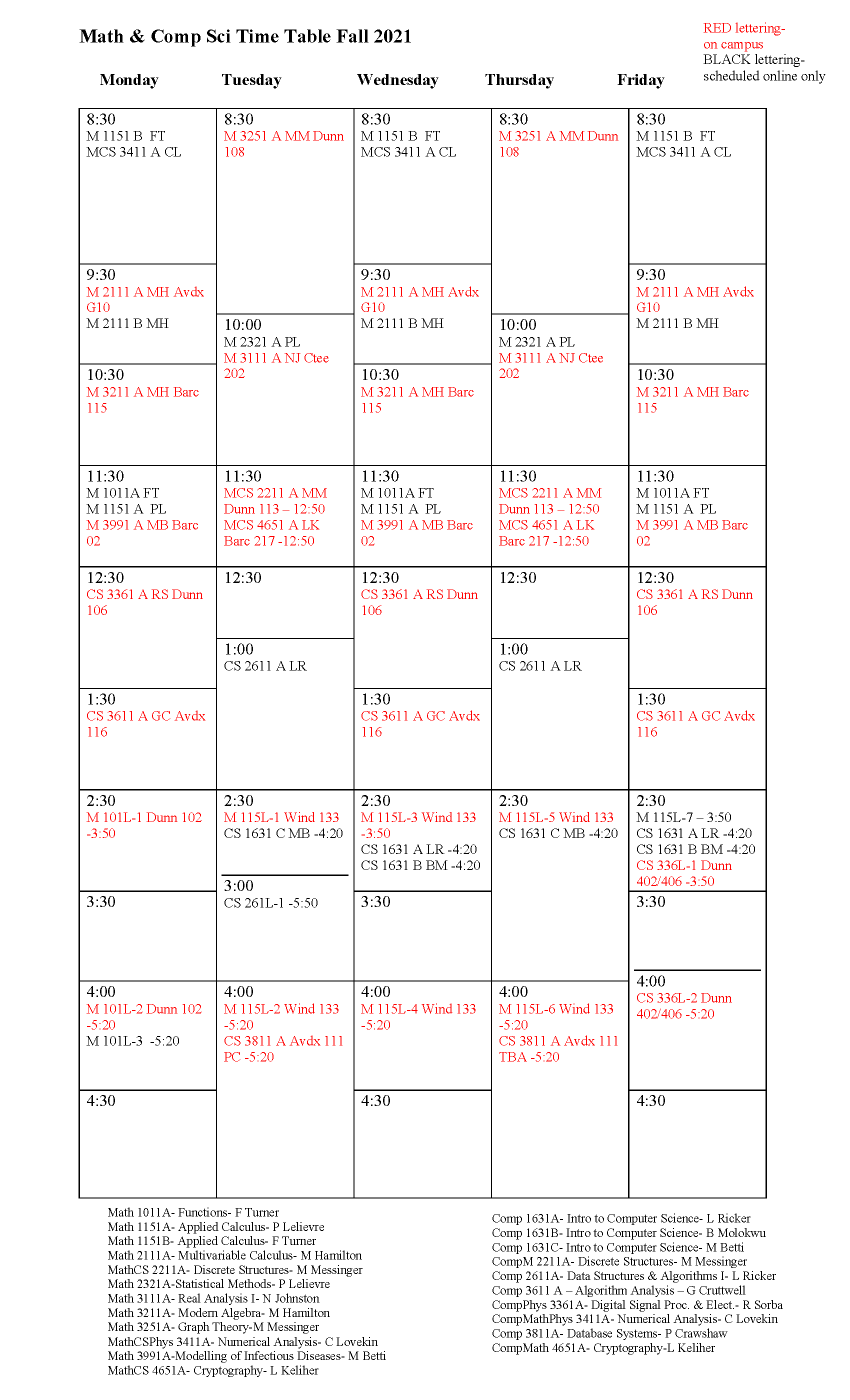## Programs

The academic calendar lists specific degree requirements for various programs.

SPECIAL TOPIC COURSE FALL 2021

MATH 3991 - Mathematical Modeling of Infectious Diseases

A lot of public health media releases speak of ‘what the models are showing’. What are these models? How are they made? Can we trust them? In this course we will explore answers to these questions through study of different types of mathematical models of disease progression. We will explore and discuss questions that mathematical models are good at answering, and what kind of questions are they not suited to.

Prerequisites: MATH 1121 and MATH 2221.

[Note: MATH 2121 and credit in a Statistics course are also highly recommended.]

GeoComputing

Geocomputing is an exciting new interdisciplinary BA program being offered at Mount Allison. Geocomputing is a joint major BA program that will give students expertise to study and understand spatial dimensions of social and environmental problems.  It is an applied program that combines theory and practice of computing and geography, and offers students a new way to look at the world.

Interest in computer science has increased in recent years, with many students hoping to use their computer science skills to enhance another area in which they are interested.  Geocomputing offers students a unique way to combine geographical and environmental skills with training in computer science.

## Sequence of upper-year courses

The following is the intended sequence of upper year course offerings for the next two years. Note that many factors could change this plan slightly.

Mathematics

#### 2021 – 2022

Math 3031 History of Mathematics
Math 3161 Complex Variables
Math 3211 Modern Algebra I
Math 3251 Graph Theory
Math/Econ 3301 Game Theory
Math/Comp 3411 Numerical    Analysis
Math/Phys 3451 Math Methods for Physics
Math 3991 Modelling of infectious diseases
Math 4111 Topology
Math 4221 Modern Algebra II
Math/Comp 4651 Cryptography

#### 2022 – 2023

2022 – 2023
Math 3011 Set Theory and Mathematical Logic
Math 3141 Vector Calculus
Math 3131 Differential Equations II
Math 3211 Modern Algebra I
Math 3231 Number Theory
Math/Econ 3301 Game Theory
Math 3311 Probability and Statistics I
Math/Phys 3451 Math Methods for Physics
Math/Comp 4631 Theory of Computation

Computer science

#### 2021 – 2022

Comp/Phys 3361 Digital Signal Processing

Comp/Math 3411 Numerical Analysis

Comp 3611 Algorithm Analysis

Comp 3811 Database Systems

Comp 3851 Computers and Society

Comp 3911 Operating Systems

Comp/Math 4651 Cryptography

Comp 4911 Computer Networks

#### 2022 – 2023

Comp 3611 Algorithm Analysis

Comp 3651 Artificial Intelligence

Comp 3711 Principles of Programming Languages

Comp 3721 Object –Oriented Design

Comp 3971 Computer Organization and Architecture

Comp/Math 4631 Theory of Computation

Comp 4721 Software Design

## Registering for calculus

2021 onward

• MATH 1151 (Applied Calculus) will be offered
• MATH 1111 (Calculus I) will not be offered

Students may use MATH 1151 as a prerequisite for MATH 1121 (Calculus II) any MtA program that currently requires MATH 1111 has agreed to accept MATH 1151 in its place.

MATH 1151-A and 1151-B will have online lectures (due to COVID-19 room capacity restrictions). Students are also required to register for a lab section. Lab section 115L-7 is the ONLY lab being offered online. All other labs will be in-person on campus. See schedule below.

Math 1151 labs Fall 2021

In-person labs

• 115L-1 (T 2:30-3:50)
• 115L-2 (T 4:00-5:20)
• 115L-3 (W 2:30-3:50)
• 115L-4 (W 4:00-5:20)
• 115L-5 (Th 2:30-3:50)
• 115L-6 (Th 4:00-5:20)

Scheduled Online lab

• 115L-7 (Fr 2:30-3:50)

Math Assessment Test

Students registering in MATH 1151 are required to pass a Math Assessment Test (each student has two attempts).

We encourage students to complete the Math Assessment Test online during the summer.  Generally, students who struggle with the Math Assessment Test, also struggle with calculus. Students who do not pass the Math Assessment Test after two attempts can take MATH 1011  to help prepare them for success in calculus.